Polynomial Multiplication Next  |  Prev  |  Up  |  Top  |  Index  |  JOS Index  |  JOS Pubs  |  JOS Home  |  Search

#### Polynomial Multiplication

Note that when you multiply two polynomials together, their coefficients are convolved. To see this, let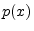denote the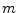th-order polynomial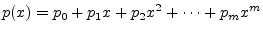with coefficients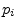, and let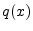denote the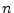th-order polynomial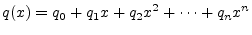with coefficients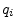. Then we have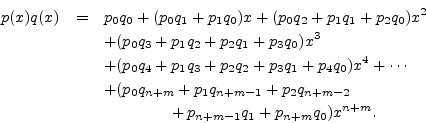Denotingby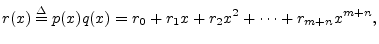we have that theth coefficient can be expressed as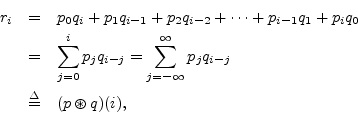whereandare doubly infinite sequences, defined as zero for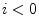and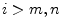, respectively.

Next  |  Prev  |  Up  |  Top  |  Index  |  JOS Index  |  JOS Pubs  |  JOS Home  |  Search

[How to cite this work] [Order a printed hardcopy]